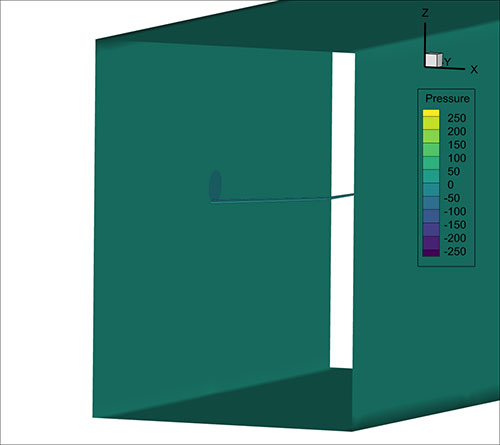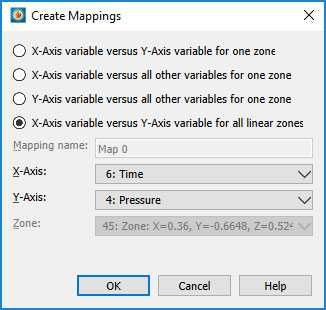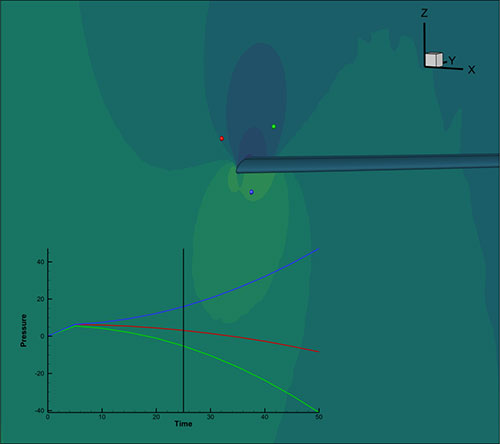# How to Extract Data from a Surface, through Time

## Extracting data from a surface, through time, using PyTecplot

In this example we want to extract Pressure values through time from the wall of a simulation at specific XYZ locations. After the extractions, we want to plot the results with Time on the X-Axis and Pressure on the Y-Axis for each XYZ location.

To do this we must use the PyTecplot function tecplot.data.probe_on_surface(). Another probe function, tecplot.data.probe_at_position(), is available but is likely to fail since it expects data to exist at the exact XYZ location (rather than near the location). Since a surface is infinitely thin it’s unlikely that the supplied XYZ location will be exactly on the surface, so we use the tecplot.data.probe_on_surface() function to find the surface value closest to the XYZ location.

### Extract Data Instructions:Figure 1. View of the wing inside the boundary

1. Load data.szplt and switch to the 3D Cartesian plot type.
2. Activate the ‘wall’ zone in the Zone Style dialog.
3. Turn on the Contour zone layer and change the contouring variable to Pressure.
4. Fit the data (Ctrl+F) and rotate the view as seen in Figure 1.
5. Allow Python connections via Scripting>PyTecplot Connections…
6. From a command prompt, ‘cd’ to the directory that contains ‘probe_on_surface_through_time.py’ and run the script using the command:
> python –O probe_on_surface_through_time.py
7. The script extracts data from the XYZ locations specified in XYZ.txt and will create one zone for each XYZ location. These zones represent the Pressure values on the wall at each of those locations.
8. To plot the new data, create a new frame and switch to XY Line plot type. Create line mappings using the Create Mappings dialog (see Figure 2 below).
9. To show the timing marker in the XY plot, run the show_markergridline.mcr macro.
10. In the 3D plot, activate the three new zones and use the Scatter plot layer to display the XYZ locations in the 3D plot.
11. The final result is shown in Figure 4.Figure 2. Create Mappings dialogFigure 3. Pressure over time at each of the three XYZ locations on the wall

### Final Result

This Tecpot image shows the line data and the XYZ locations in context of the 3D plot.Figure 4. Final result showing the line data and the XYZ locations in context of the 3D plot

Also see the related Tecplot 360 Video Tutorial, External Flow – Comparing a CFD Simulation with Experimental Data.